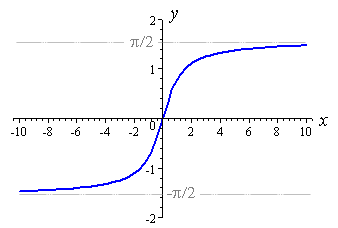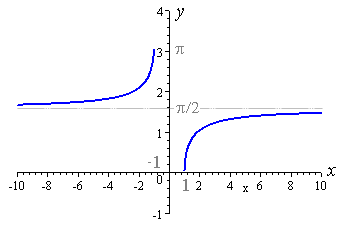# Trig Properties

The flashcards below were created by user ffarouk on FreezingBlue Flashcards.

1. tan
1/cot or sin/cos
2. sec
1/cos
3. csc
1/sin
4. cot
cos/sin
5. tan, sin, csc, cot
Odd trig finction with pi(k),
6. cos, sec
Even trig functions with 2(pi)(k)
7. Graph: sin
Domain: all real numbers

Range: -1, 1 (closed)8. Graph: cos
Domain: all real numbers

Range: -1,1 (closed)9. Graph: csc
Domain: all real numbers, expect that theta = (pi)k; k any integer

Range: -infinity (open) to -1 (close) Union 1 (closed) to +infinity (open)10. Graph: sec
Domain: all real numbers, expect that theta = (pi/2)k; k any odd integer

Range: -infinity (open) to -1 (close) Union 1 (closed) to +infinity (open)11. Graph: tan
Domain: all real numbers, expect that theta = (pi/2)k; k any odd integer

Range: all real numbers12. Gaph: cot
Domain: all real numbers, expect that theta = (pi)k; k an integer

Range: all real numbers13. Graph: sin-1
Domain: [-1 , 1]

Range: [-pi/2 , pi2]14. Graph: cos-1
Domain: [-1 , 1]

Range: [0 , pi]15. Graph: tan-1
Domain: all real numbers

Range: ( -pi/2 , pi/2)16. Graph: cot-1
Domain: all real numbers

Range: (0 , pi)17. Graph: sec-1
Domain: (-infinity , -1] U [1 , +infinity)

Range: [0 , pi] NOT including pi/218. Graph: csc-1
Domain: (-infinity , -1] U [1 , +infinity]

Range: [-pi/2 , pi/2] NOT including 019. Double Angle Foumula:

sin(2x) =
2sin(x)cos(x)
20. Double Angle Formula:

cos2(x) - sin2(x)
2cos2(x) - 1
21. Derivative: sin(x)
cos(x)
22. Derivative: cos(x)
-sin(x)
23. Derivative: tan(x)
sec2(x)
24. Derivative: cot(x)
-csc2(x)
25. Derivative: sec(x)
sec(x)tan(x)
26. Derivative: csc(x)
-csc(x)cot(x)
27. Double Angle Formula:

cos(2x)
1-2sin2(x)
 Author: ffarouk ID: 41744 Card Set: Trig Properties Updated: 2010-10-17 20:54:30 Tags: QBIC Calculus trig Folders: Description: Tirg Properties Show Answers: